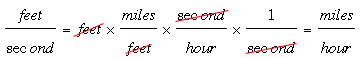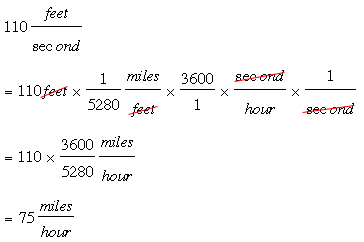SEARCH HOMEMath Central Quandaries & QueriesQuestion from jacob, a student: If it took an ostrich 2.5 seconds to travel 110 feet and the average speed is 44 feet/ seconds, what was the ostrich's speed in miles per hour?Hi Jacob,

A cheetah can run as fast as 110 ft/sec. If I want that in miles per hour then the units tell me what to doThere are 5280 feet in a mile and 60 × 60 = 3600 seconds in an hour so lets fill in the numbersNow try the ostrich speed.

PennyMath Central is supported by the University of Regina and The Pacific Institute for the Mathematical Sciences.